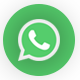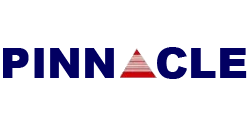# Reasoning Practice Set Paper For SSC CGL Exam Tier 1

Validity: 9 Months
What you will get
Course Highlights
• Based on latest Pattern
• English Medium eBooks
SSC

# Reasoning Practice Set Paper For SSC CGL Exam Tier 1

Reasoning Practice Set Paper For SSC CGL Exam Tier 1

Q1. If FRIEND  is coded  as HUMJTK , how can CANDLE can be written in that code ?

(a) EDRIRL

(b) ESJFME

(c) DCQHQK

(d) DEQJQM

Ans. (a)

Q2. If A denotes - ,   C denotes × , D denotes ÷ , E denotes + , then 14C3A12E4D2=?

(a) 6

(b) 17

(c) 28

(d) 32

Ans. (d)

Q3. If 879 =8,  625=1, 586=9,

Then 758=?

(a) 6

(b) 7

(c) 8

(d) 9

Ans. (a)

Q4. Find the  missing numbers from the  given alternatives :(a) 54

(b) 44

(c) 34

(d) 26

Ans. (b)

Q5. Sham goes  to his friends house  that is straight 10 Km from his house .on his  way  back,he takes right turn  and walks 2 KM again takes a left  turn   and walks  of 10 KM.  How much distance  is sham still away  from his house ?

(a) 10Km.

(b) 8Km.

(c) 12Km.

(d)  2Km.

Ans. (d)

Q6. One or two statement are Given followed by two conclusions , I and II. You have to consider  the statement is true , if it seem to be at variance from commonly  know facts . you are to decide which of the  given  conclusions /assumptions can definitely be drawn from the given statement . indicate your answer .

Statement : All boys are tall. Rajiv is a boy.

Conclusions

I : Rajiv is tall.

II : Rajiv is not tall.

(a) Only conclusions I  follows

(b) Only conclusions II  follows

(c) Both conclusion I and II Conclusions follow

(d) Neither Conclusion I nor conclusions II follow

Ans. (a)

Q7. How many  Triangles  are there in the questions Figure .(a) 6

(b) 10

(c) 12

(d) 16

Ans. (d)

Q8. Which of the following diagram Represent  the  following  relationship between

Awards , Pulitzer and Prize Oscars.

(a)(b)(c)(d)Ans. (c)

Q9. Which answer  Figure will complete the pattern in the question figure .(a)(b)(c)(d)Ans. (c)

Q10. From the given answer figures , select the one in which the question figure is hidden

embedded .(a)(b)(c)(d)Ans. (b)

Q11. A piece of paper is folded and  cut as show below in the questions figure from the given answer Figure  ,indicate how it will appear when opened .(a)(b)(c)(d)Ans. (d)

Q12. If a mirror  is placed on the line MN , then which of the answer Figure is the right images of the given figure?(a)(b)(c)(d)Ans. (b)